SLES102B December   2003  – March 2015

PRODUCTION DATA.

1. Features
2. Applications
3. Description
4. Revision History
5. Pin Configuration and Functions
6. Specifications
7. Detailed Description
1. 7.1 Overview
2. 7.2 Functional Block Diagram
3. 7.3 Feature Description
1. 7.3.1 System Clock and Reset Functions
2. 7.3.2 Power-On and External Reset Functions
3. 7.3.3 Audio Data Interface
4. 7.3.4 Function Descriptions
4. 7.4 Device Functional Modes
8. Application and Implementation
1. 8.1 Application Information
2. 8.2 Typical Applications
1. 8.2.1 Application for External Digital Filter Interface
2. 8.2.2 PCM1798 Typical Application
1. 8.2.2.1 Design Requirements
2. 8.2.2.2 Detailed Design Procedure
9. Power Supply Recommendations
10. 10Layout
11. 11Device and Documentation Support
12. 12Mechanical, Packaging, and Orderable Information

#### Package Options

Refer to the PDF data sheet for device specific package drawings

• DB|28

## 6 Specifications

### 6.1 Absolute Maximum Ratings

over operating free-air temperature range (unless otherwise noted) (1)
MIN MAX UNIT
Supply voltage VCC1, VCC2L, VCC2R –0.3 6.5 V
VDD –0.3 4
Supply voltage differences: VCC1, VCC2L, VCC2R ±0.1 V
Ground voltage differences: AGND1, AGND2, AGND3L, AGND3R, DGND ±0.1 V
Digital input voltage LRCK, DATA, BCK, SCK, FMT1, FMT0, MONO, CHSL, DEM, MUTE, RST –0.3 6.5 V
ZERO –0.3 (VDD + 0.3 V) < 4
Input current (any pins except supplies) ±10 mA
Ambient temperature under bias –40 125 °C
Junction temperature 150 °C
Package temperature (IR reflow, peak) 260 °C
Storage temperature, Tstg –55 150 °C
(1) Stresses beyond those listed under Absolute Maximum Ratings may cause permanent damage to the device. These are stress ratings only, which do not imply functional operation of the device at these or any other conditions beyond those indicated under Recommended Operating Conditions. Exposure to absolute-maximum-rated conditions for extended periods may affect device reliability.

### 6.2 ESD Ratings

VALUE UNIT
V(ESD) Electrostatic discharge Human body model (HBM), per ANSI/ESDA/JEDEC JS-001(1) ±3000 V
Charged-device model (CDM), per JEDEC specification JESD22-C101(2) ±1500
(1) JEDEC document JEP155 states that 500-V HBM allows safe manufacturing with a standard ESD control process.
(2) JEDEC document JEP157 states that 250-V CDM allows safe manufacturing with a standard ESD control process.

### 6.3 Recommended Operating Conditions

over operating free-air temperature range (unless otherwise noted)
MIN NOM MAX UNIT
VDD Digital supply voltage 3.0 3.3 3.6 V
VCC1 Analog supply voltage 4.7525 5 5.25 V
VCC2L
VCC2R
Operating temperature –25 85 °C

### 6.4 Thermal Information

THERMAL METRIC(1) PCM1798 UNIT
DB (SSOP)
28 PINS
RθJA Junction-to-ambient thermal resistance 70.4 °C/W
RθJC(top) Junction-to-case (top) thermal resistance 29.2
RθJB Junction-to-board thermal resistance 31.5
ψJT Junction-to-top characterization parameter 3.1
ψJB Junction-to-board characterization parameter 31.1
RθJC(bot) Junction-to-case (bottom) thermal resistance n/a

### 6.5 Electrical Characteristics

All specifications at TA = 25°C, VCC1 = VCC2L = VCC2R = 5 V, VDD = 3.3 V, fS = 44.1 kHz, system clock = 256 fS, and 24-bit data (unless otherwise noted)
PARAMETER TEST CONDITIONS MIN TYP MAX UNIT
Resolution 24 Bits
DATA FORMAT
Audio data interface format Standard, I2S, left-justified
Audio data bit length 16-, 24-bit selectable
Audio data format MSB first, 2s complement
fS Sampling frequency 10 200 kHz
System clock frequency 128, 192, 256, 384, 512, 768 fS
DIGITAL INPUT/OUTPUT
Logic family TTL compatible
VIH Input logic level 2 VDC
VIL 0.8
IIH Input logic current VIN = VDD 10 µA
IIL VIN = 0 V –10
VOH Output logic level IOH = –2 mA 2.4 VDC
VOL IOL = 2 mA 0.4
DYNAMIC PERFORMANCE(1)(2)
THD+N at VOUT = 0 dB fS = 44.1 kHz 0.0005% 0.001%
fS = 96 kHz 0.00%
fS = 192 kHz 0.0015%
Dynamic range EIAJ, A-weighted, fS = 44.1 kHz 120 123 dB
EIAJ, A-weighted, fS = 96 kHz 123
EIAJ, A-weighted, fS = 192 kHz 123
Signal-to-noise ratio EIAJ, A-weighted, fS = 44.1 kHz 120 123 dB
EIAJ, A-weighted, fS = 96 kHz 123
EIAJ, A-weighted, fS = 192 kHz 123
Channel separation fS = 44.1 kHz 116 119 dB
fS = 96 kHz 118
fS = 192 kHz 117
Level linearity error VOUT = –120 dB ±1 dB
DYNAMIC PERFORMANCE (MONO MODE)(1)(2)(3)
THD+N at VOUT = 0 dB fS = 44.1 kHz 0.0005%
fS = 96 kHz 0.001%
fS = 192 kHz 0.0015%
Dynamic range EIAJ, A-weighted, fS = 44.1 kHz 126 dB
EIAJ, A-weighted, fS = 96 kHz 126
EIAJ, A-weighted, fS = 192 kHz 126
Signal-to-noise ratio EIAJ, A-weighted, fS = 44.1 kHz 126 dB
EIAJ, A-weighted, fS = 96 kHz 126
EIAJ, A-weighted, fS = 192 kHz 126
ANALOG OUTPUT
Gain error –7 ±2 7 % of FSR
Gain mismatch, channel-to-channel –3 ±0.5 3 % of FSR
Bipolar zero error At BPZ –2 ±0.5 2 % of FSR
Output current Full scale (0 dB) 4 mAp-p
Center current At BPZ –3.5 mA
DIGITAL FILTER PERFORMANCE
De-emphasis error ±0.1 dB
FILTER CHARACTERISTICS–1: SHARP ROLLOFF
±0.0002 dB 0.454 fS
Pass band –3 dB 0.49 fS
Stop band 0.546 fS
Pass-band ripple ±0.0002 dB
Stop-band attenuation Stop band = 0.546 fS –98 dB
Delay time 38/fS s
FILTER CHARACTERISTICS–2: SLOW ROLLOFF
Pass band ±0.001 dB 0.21 fS
–3 dB 0.448 fS
Stop band 0.79 fS
Pass-band ripple ±0.001 dB
Stop-band attenuation Stop band = 0.732 fS –80 dB
Delay time 38/fS s
POWER SUPPLY REQUIREMENTS
VDD Voltage range 36 3.3 3.6 VDC
VCC1
VCC2L 4.7525 5 5.25
VCC2R
IDD Supply current(4) fS = 44.1 kHz 7 9 mA
fS = 96 kHz 13
fS = 192 kHz 25
ICC fS = 44.1 kHz 18 23 mA
fS = 96 kHz 19
fS = 192 kHz 20
Power dissipation(4) fS = 44.1 kHz 115 150 mW
fS = 96 kHz 140
fS = 192 kHz 180
TEMPERATURE RANGE
Operation temperature –25 85 °C
(1) Filter conditions:
THD+N: 20-Hz HPF, 20-kHz AES17 LPF
Dynamic range: 20-Hz HPF, 20-kHz AES17 LPF, A-weighted
Signal-to-noise ratio: 20-Hz HPF, 20-kHz AES17 LPF, A-weighted
Channel separation: 20-Hz HPF, 20-kHz AES17 LPF
Analog performance specifications are measured using the System Two™ Cascade audio measurement system by Audio Precision™ in the averaging mode.
(2) Dynamic performance and dc accuracy are specified at the output of the post amplifier as shown in Figure 32.
(3) Dynamic performance and dc accuracy are specified at the output of the measurement circuit as shown in Figure 33.
(4) Input is BPZ data.

### 6.6 Timing Requirements

MIN MAX UNIT
SYSTEM CLOCK INPUT TIMING
t(SCY) System clock pulse cycle time 13 ns
t(SCKH) System clock pulse duration, HIGH 0.4t(SCY) ns
t(SCKL) System clock pulse duration, LOW 0.4t(SCY) ns
EXTERNAL RESET TIMING
t(RST) Reset pulse duration, Low 20 ns
TIMING OF AUDIO INTERFACE
t(BCY) BCK pulse cycle time 70 ns
t(BCL) BCK pulse duration, LOW 30 ns
t(BCH) BCK pulse duration, HIGH 30 ns
t(BL) BCK rising edge to LRCK edge 10 ns
t(LB) LRCK edge to BCK rising edge 10 ns
t(DS) DATA setup time 10 ns
t(DH) DATA hold time 10 ns
LRCK clock data 50% ± 2 bit clocks
AUDIO INTERFACE TIMING FOR EXTERNAL DIGITAL FILTER
t(BCY) BCK pulse cycle time 20 ns
t(BCL) BCK pulse duration, LOW 7 ns
t(BCH) BCK pulse duration, HIGH 7 ns
t(BL) BCK rising edge to WDCK falling edge 5 ns
t(LB) WDCK falling edge to BCK rising edge 5 ns
t(DS) DATA setup time 5 ns
t(DH) DATA hold time 5 ns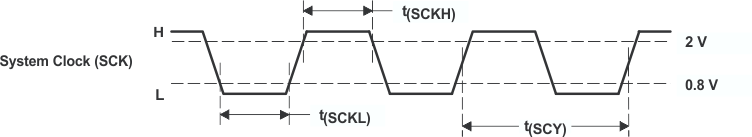Figure 1. System Clock Input Timing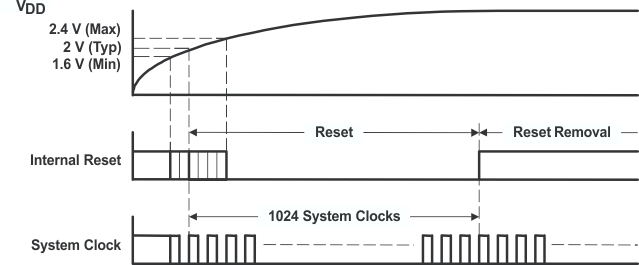Figure 2. Power-On Reset Timing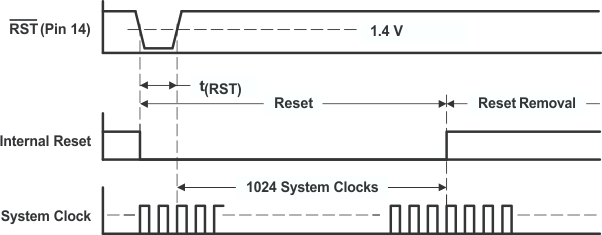Figure 3. External Reset Timing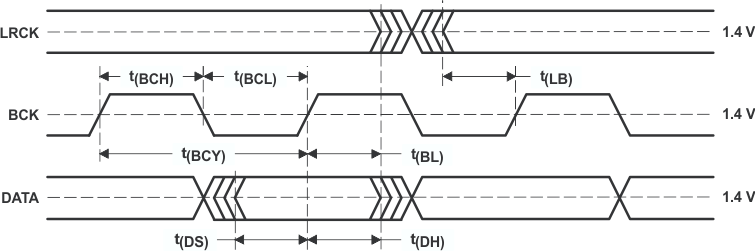Figure 4. Timing of Audio Interface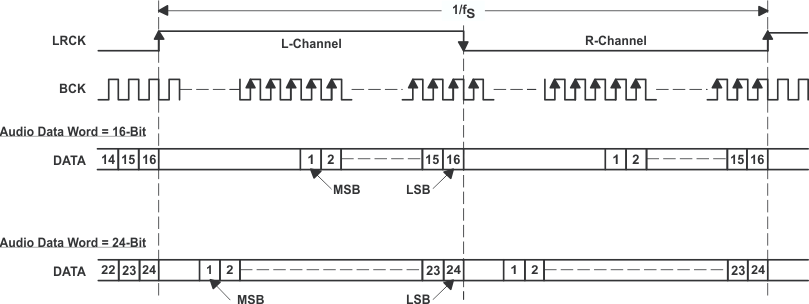(1) Standard Data Format (Right-Justified); L-Channel = HIGH, R-Channel = LOW
Figure 5. Auto Data Input Format (1 of 3)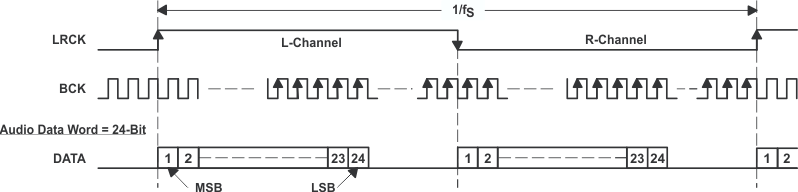(2) Left-Justified Data Format; L-Channel = HIGH, R-Channel = LOW
Figure 6. Auto Data Input Format (2 of 3)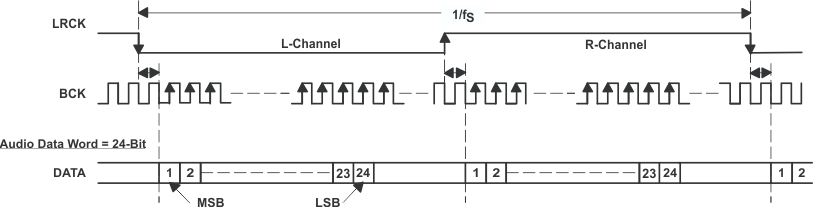(3) I2S Data Format; L-Channel = LOW, R-Channel = HIGH
Figure 7. Auto Data Input Format (3 of 3)Figure 8. Audio Interface Timing for External Digital Filter (Internal DF Bypass Mode) Application

### 6.7 Typical Characteristics for Digital Filter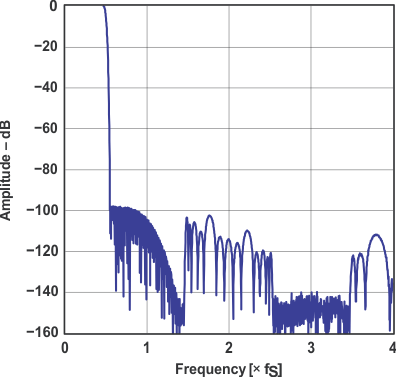Frequency Response, Sharp Rolloff
Figure 9. Amplitude vs Frequency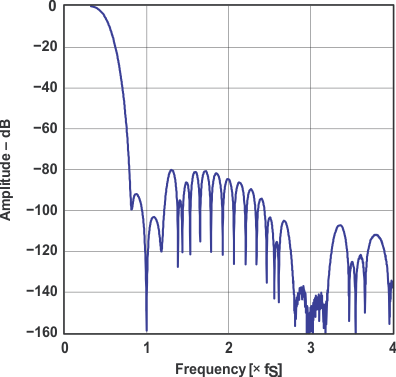Frequency Response, Slow Rolloff
Figure 11. Amplitude vs Frequency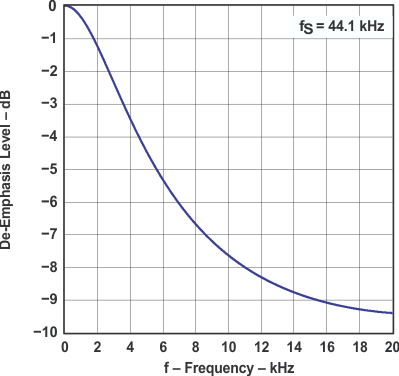Figure 13. De-emphasis Level vs Frequency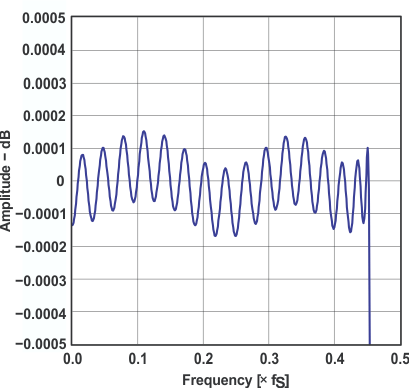Pass-Band Ripple, Sharp Rolloff
Figure 10. Amplitude vs Frequency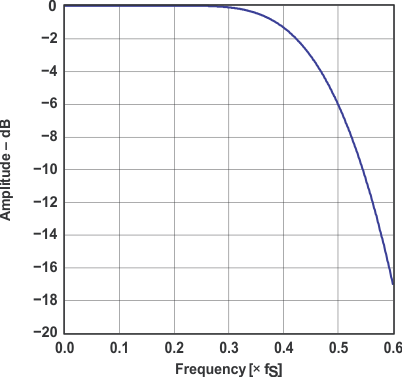Transition Characteristics, Slow Rolloff
Figure 12. Amplitude vs FrequencyFigure 14. De-emphasis Error vs Frequency

#### 6.7.1 Analog Dynamic Performance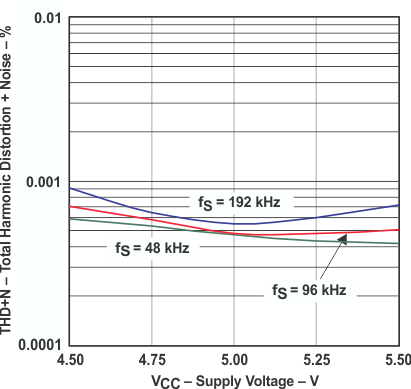NOTE: PCM mode, TA = 25°C, VDD = 3.3 V, measurement circuit is Figure 32.
Figure 15. Total Harmonic Distortion + Noise
vs Supply Voltage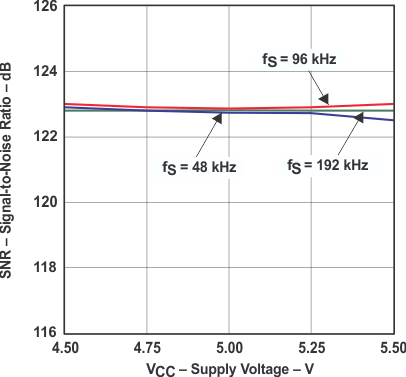NOTE: PCM mode, TA = 25°C, VDD = 3.3 V, measurement circuit is Figure 32.
Figure 17. Signal-to-Noise Ratio vs Supply Voltage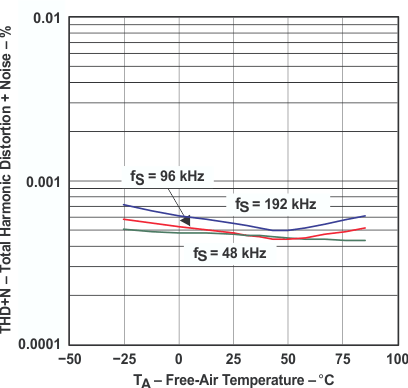NOTE: PCM mode, VDD = 3.3 V, VCC = 5 V, measurement circuit is Figure 32.
Figure 19. Total Harmonic Distortion + Noise
vs Free-air TemperatureNOTE: PCM mode, VDD = 3.3 V, VCC = 5 V, measurement circuit is Figure 32.
Figure 21. Signal-to-noise Ratio vs Free-air Temperature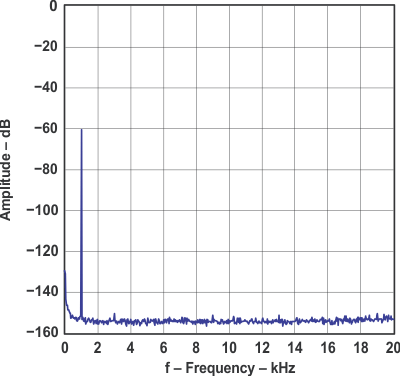NOTE: fS = 48 kHz, 32768 point 8 average, TA = 25°C, VDD = 3.3 V, VCC = 5 V, measurement circuit is Figure 32.
Figure 23. Amplitude vs Frequency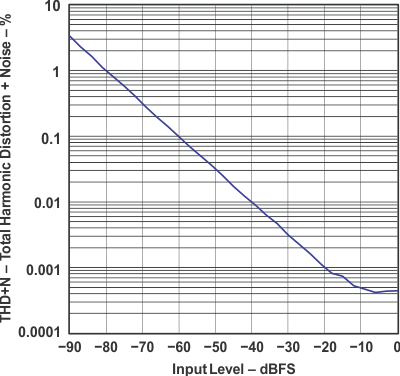NOTE: fS = 48 kHz, TA = 25°C, VDD = 3.3 V, VCC = 5 V, measurement circuit is Figure 32.
Figure 25. Total Harmonic Distortion + Noise vs Input LevelNOTE: PCM mode, TA = 25°C, VDD = 3.3 V, measurement circuit is Figure 32.
Figure 16. Dynamic Range vs Supply Voltage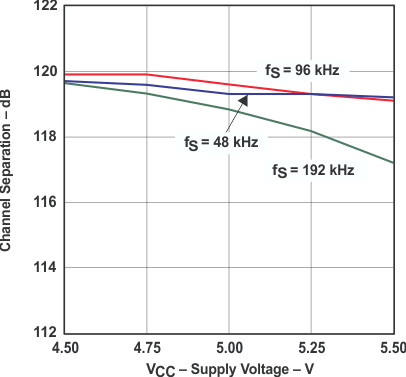NOTE: PCM mode, TA = 25°C, VDD = 3.3 V, measurement circuit is Figure 32.
Figure 18. Channel Separation vs Supply Voltage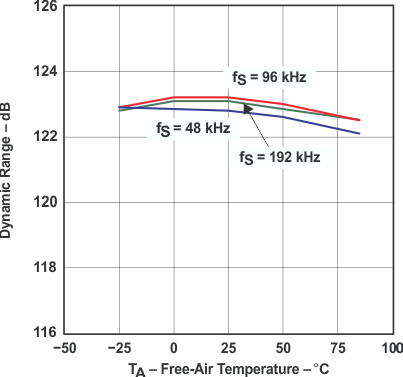NOTE: PCM mode, VDD = 3.3 V, VCC = 5 V, measurement circuit is Figure 32.
Figure 20. Dynamic Range vs Free-air Temperature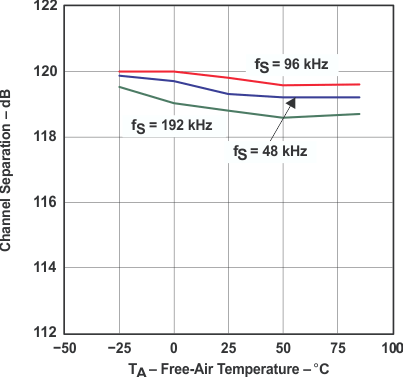NOTE: PCM mode, VDD = 3.3 V, VCC = 5 V, measurement circuit is Figure 32.
Figure 22. Channel Separation vs Free-air Temperature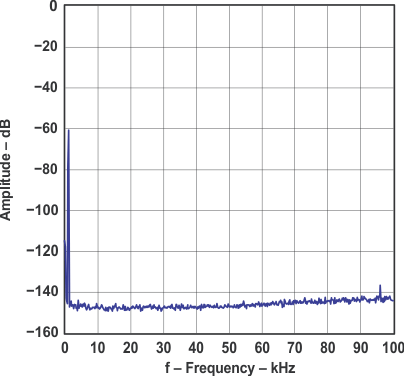NOTE: fS = 96 kHz, 32768 point 8 average, TA = 25°C, VDD = 3.3 V, VCC = 5 V, measurement circuit is Figure 32.
Figure 24. Amplitude vs Frequency